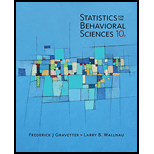Chapter 3, Problem 24PStatistics for The Behavioral Scie...

10th Edition
Frederick J Gravetter + 1 other
ISBN: 9781305504912

Solutions

Chapter
SectionStatistics for The Behavioral Scie...

10th Edition
Frederick J Gravetter + 1 other
ISBN: 9781305504912
Textbook Problem

Schmidt (1994) conducted a series of experiments examining the effects of humor on memory. In one study, participants were shown a list of sentences, of which half were humorous and half were non humorous. A humorous example is, "If at first you don't succeed, you are probably not related to the boss." Other participants would see a nonhumorous version of this sentence, such as "People who are related to the boss often succeed the very first time." Schmidt then measured the number of each type of sentence recalled by each participant. The following scores are similar to the results obtained in the study. Number of Sentence Recalled Humorous NonhumorousSentencesSentences 4 5 2 4 5 2 4 2 6 7 6 6 2 3 l 6 2 5 4 3 3 2 3 3 1 3 :5 5 4 1 5 3 Calculate the mean number of sentences recalled for each of the two conditions. Do the data suggest that humor help memory'?

To determine

Find the mean for the given sample of scores.

Explanation

The mean is the average of scores. To find mean, add all scores and divide the sum by number of scores in the sample.

Given:

The sample of scores:

 Humorous Sentences 4 5 2 4 6 7 6 6 2 5 4 3 1 3 5 5 Non-humorous Sentences 5 2 4 2 2 3 1 6 3 2 3 3 4 1 5 3

Formula used:

M= x n

Calculations:

To find mean, add all scores and divide the sum by number of scores in the sample

Still sussing out bartleby?

Check out a sample textbook solution.

See a sample solution

The Solution to Your Study Problems

Bartleby provides explanations to thousands of textbook problems written by our experts, many with advanced degrees!

Get Started

Find more solutions based on key concepts